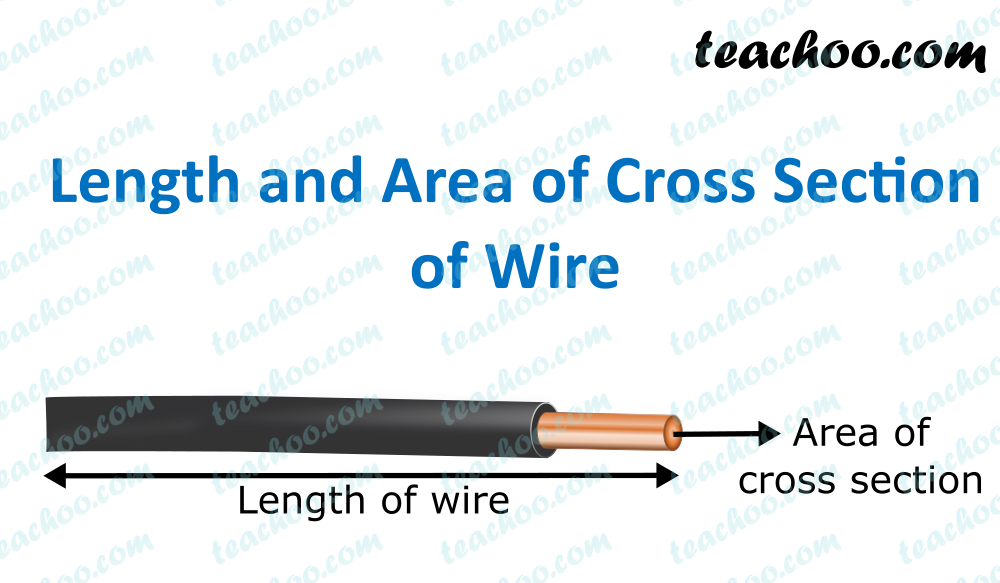Concepts

Class 10
Chapter 12 Class 10 - Electricity

It depends upon following factors

## Length of Conductor

Resistance of a conductor is directly proportional to its length

R ∝ l

if we increase the length of a wire (conductor),its resistance increases(and hence less current can pass through it)

If we reduce length,resistance decreases (and hence more current can pass through it)

## Area of Cross SectionIf we cut a wire and see through it horizontally,it is in form of a circle

This Area of Circle is called Area of Cross Section of the Wire

Resistance of a conductor is inversely proportional to its area of cross- section.

R ∝ 1/A

Suppose a wire is 14 mm thick

It means its diameter has 14 mm

Area of Cross Section of Wire= πr 2

Area of Cross Section of Wire= 22/7×7 2

Area of Cross Section of Wire= 154 m 2

If we double Area of Cross Section (by increasing thickness of wire)

Resistance gets halved and hence more electricity can pass through it.

But if we reduce area of cross section(example we take thin fire)

Resistance increases and hence less electricity can pass through it

Note - This is the reason that we use thick wire to carry more electrical load (as thick wire has more area of cross section and offers less resistance and more electricity can pass through it )

## Nature of Material

Resistance also depends on the nature of the material

Conductors offer less resistance(Example- copper)

Insulators offer more resistance (Example- Plastic)

## Effect of Temperature on Resistance

In case of Pure Metals, Resistance increases on Increasing Temperature and Decreases on Decreasing Temperature.

But in case of alloys (like nichrome), Temperature has no effect on the resistance.

Now, we know that

R ∝ l

And, R ∝ 1/A

Combining the above two, we get

R ∝ l/A

R = constant x (l/A)

R = ρ (l/A)

This constant is called resistivity.

It is denoted by ρ (rho)

We will study more about resistivity in the next post .

Questions

NCERT Question 18 (d) - How does the resistance of a wire vary with its area of cross-section?

Q1 Page 209 - On what factors does the resistance of a conductor depend?

Q2 Page 209 - Will current flow more easily through a thick wire or a thin wire of the same material, when connected to the same source? Why?

Q3 Page 209 - Let the resistance of an electrical component remains constant while the potential difference across the two ends of the component decreases to half of its former value. What change will occur in the current through it?

Question - A cylindrical conductor of length l and uniform area of cross section A has resistance R. Another conductor of length 2l and resistance R of the same material has area of cross section

(a) A/2

(b) 3A/2

(c) 2A

(d) 3A

Learn in your speed, with individual attention - Teachoo Maths 1-on-1 Class